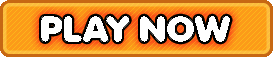Bookmark

# Fast Facts Basic Math Facts Addition, Subtraction, Multiplication, Division

5 stars (738 plays / 1 votes)Fast Facts | Basic Math Facts (Addition, Subtraction, Multiplication, & Division)How quickly can you correctly answer 10 math facts?
5 stars out of 5 (738 plays / 1 votes)

KidzSearch > Games > Math GamesTags:  math    basic    fact    addition    subtraction    multiplication    division    problem    elementary    math    number    sense    first    timed    test    quiz    divide    multiplyMember of the American Library Association  Copyright 2005-2020 KidzSearch.com